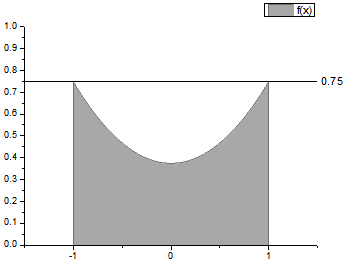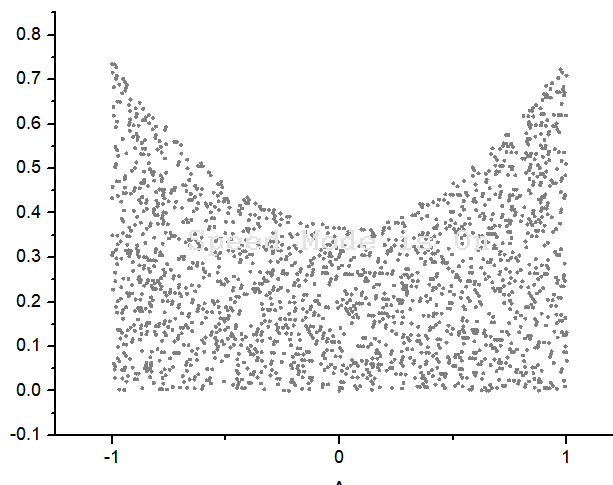# Acceptance-Rejection Method (Rejection Sampling) for generating distributions- C PROGRAM

Acceptance-Rejection method can be used to produce random numbers following a certain probability density function.

This is done by generating random numbers following a uniform distribution and then rejecting those that don’t follow the desired distribution. For example: Let’ say you have generate random nos. following the probability density function,$f(x)$ where$x_{min} \leq x \leq x_{max}$

Then, the procedure would be:

1. Generate uniformly distributed random nos.$X$ b/w$x_{min}$ and$x_{max}$.
2. Generate uniformly distributed random nos.$Y$ b/w$0$ &$f_{max}$.
3. If$Y \leq f(X)$ then accept$X$ and$Y$.
4. You can plot the accepted$X$ and$Y$, to see that they follow the required distribution.

Note: For step 2. you would need to find out the maximum value of the desired pdf for a given range of x.
Then to generate Y (random nos. b/w 0 and fmax), just generate uniformly distributed random nos. b/w 0 and 1 and multiply them by fmax.
Similarly for step 1: X can be generated by generating uniformly distributed random nos. from 0 to m using any of the previously discussed techniques and then using the following relation:

Let’s say we require random nos. with the pdf$f(x)=\frac{3}{8}(1+x^2)$ for$-1 \leq x \leq 1$. For the given range of x, clearly$f_{max}=3/4$.

Now an intuitive way to look at the above algorithm is, that when we generate X and Y, we are in fact picking the point (X,Y) in the rectangular box below. And the test in step 3. ensures that point lies below the graph of f(x).
It seems plausible that if we keep only the points that fall under the graph of the density f(x), and ignore the points above, then the distribution of the abscissa should have density f(x).Generate (X,Y) within the rectangle and then select those that lie below the f(x) curve

The following C program illustrates the entire procedure for the above example.

### CODE:

/******************************************************
*************ACCEPTANCE-REJECTION PROBLEM**************
******************************************************/
#include<stdio.h>
#include<math.h>
/**
Probabitlity distribution function acc. to which the random nos. are required
**/
double f(double x){
return 3/8.0*(1+x*x);
}
/**Function that generates a random number.
Parameters:
r0: initial (first) seed
a: scale factor , so that a*r0 give the first random number
m: gives the max. value of random numbers that can be generated (m-1)
c: additional displacement(offset) factor
**/
int rand(int r0, int a, int m, int c){
double r1=(a*r0+c)%m;
return r1;
}
/**Function that generates random numbers given a seed, and stores them in an array that is passed as an argument.
Parameters:
r0: initial (first) seed
a: scale factor , so that a*r0 give the first random number
m: gives the max. value of random numbers that can be generated (m-1)
c: additional displacement factor
n: no. of random numbers to be generated
x[n]: array that will store the random numbers
**/
void randomNos(int r0, int a, int m, int c, int n, int x[n]){
double r1=rand(r0,a,m,c);
int i;
for(i=0;i<n;i++){
x[i]=r1;
r1=rand(r1,a,m,c);
}
}
/**Function that generates random numbers in a given range: [min,max], given a seed r0, and stores them in an array that is passed as an argument.
Parameters:
r0: initial (first) seed
a: scale factor , so that a*r0 give the first random number
m: gives the max. value of random numbers that can be generated (m-1)
c: additional displacement factor
n: no. of random numbers to be generated
x[n]: array that will store the random numbers
min: lower limit for random nos.
max: upper limit for random nos.
**/
void randomNosRange(int n, double r[n], double x[n], double min, double max){
int i;
double r1;
for(i=0;i<n;i++){
r1=min+(max-min)*r[i];
x[i]=r1;
}
}
main(){
int min=-1, max=1, a=1093, m=86436, c=18257, M=10;
double fmax=3/4.0;  //Max value of the function
int n=35000;
int i,j;
int rand01[n];		//for  n Random Nos from 0 to 86435
int rand02[n];		//for  n Random Nos from 0 to 86435
double r1[n];		//for  n Random Nos from 0 to 1
double r2[n];		//for  n Random Nos from 0 to 1
double x[n];		//for  n Random Nos from min to max
randomNos(43,a,m,c,n,rand01);		//gives  n Random Nos from 0 to 86435 and stores them in rand01
randomNos(23,a,m,c,n,rand02);		//gives  n Random Nos from 0 to 86435 and stores them in rand02
//Normalize random nos. in rand01 to [0,1] range and store them r1
for(i=0;i<n;i++){
r1[i]=(double)rand01[i]/(m-1);
}
//convert the random nos. in r1 in the range [0,1] to random nos. in the range [min,max] and store them in x array
randomNosRange(n,r1,x,min,max);
//Normalize random nos. in rand02 to [0,1] range and store them r2
for(i=0;i<n;i++){
r2[i]=(double)rand02[i]/(m-1);
}
double y[n];	//for n random nos from 0 to fmax
for(i=0;i<n;i++){
y[i]=r2[i]*fmax;	//get random nos from 0 to fmax in y array
}
//an array to keep track of the random nos lying below the given function
int Naccept=0;
//Arrays that will store the x and y values that are accepted that is lie below the given funvtion f
double xAccept[n];
double yAccept[n];
//Begin acceptance-rejection
for(i=0;i<n;i++){

if(y[i]<=f(x[i])){
Naccept++;
xAccept[i]=x[i];
yAccept[i]=y[i];
}
}
FILE *fp=NULL;
fp=fopen("acceptRejectProb.txt","w");
//Store the accepted X and Y in a file
for(i=0;i<Naccept;i++){
fprintf(fp,"%lf\t%lf\n",xAccept[i],yAccept[i]);
}

}


### OUTPUT:[wpedon id="7041" align="center"]

## 5 thoughts on “Acceptance-Rejection Method (Rejection Sampling) for generating distributions- C PROGRAM”

1. Hi, could I ask how the values of a, c and m are determined? Also where do the initial values 23 and 43 come from?

1. Thats a very good question. The values of a, m and c are from Numerical Recipes book. Seeds: 43 and 23 were arbitrary I guess. You can use anything there.

2. Thank you for writing this article. I have enjoyed playing around with this code. Although it works well for smaller values of n, at higher values of n you get Segmentation faults. I assume from trying to store longer arrays. The code seems quite ineffiecient storage-wise as arrays are replicated and waste data removed.

1. Hi, You’re correct that the program is inefficient and won’t work for large values of ‘n’. However, the purpose was just to do a quick demonstration rather than actually use it somewhere. The main problem is that I am using static arrays therefore they are only stored on stack. So increasing the stack size may help but again not a good solution. Ideal would be to use dynamic arrays.

1. Exactly. Dynamic Array should help.